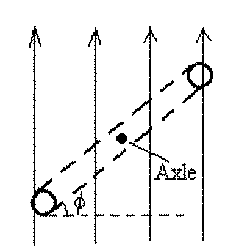## University of California, San Diego Physics 1b - Thermal Physics & Electromagnetism

 H. E. Smith Spring 2000Physics 1B - Tutorial #91. The figure shows two long current-carrying wires. What is the value of the magnetic field at point P, 1m away from the crossing point? What would be the value of the magnetic field if one of the currents were reversed?
• B = 2µ0/2r = 2 x (4x 10-7.3/ (2.0.5m) = 2.4 x 10-6T
• If one of the currents were reversed, B = 0.

2. The figure (Hecht p. 820, q. 4) shows an electric door bell. The circuit consists of a battery, a switch at the door, a "make-break" contact and an electromagnet. Explain what happens when the switch is pressed. Is this a "ding-dong" chime, a buzzer, or what?
Close the switch (push the button!) - current circulates, coils of the electromagnet create magnetic field (Which direction?) which pulls on the iron bar and strikes the bell. This also pulls the make-break contact apart, breaking the circuit and cutting off the current. This turns off the electromagnet, releasing the bar and allowing the spring to "make" the contact again. Current circulates, ... This cycle will continue repeatedly until the visitor lays off the button or th battery dies. Bell rings repeatedly, so this is not a "ding-dong" bell.3. The figure shows several different loops in magnetic fields. In each case the magnetic field is perpendicular to the plane of the loop and the field strength is indicated/ The numbers by the sides of the loops indicate the lengths of the sides. Order the loops in order of magnetic flux, from smallest to largest.
The magnetic flux is, left-to-right: 4,2,2,4,2. So loops 2, 3, & 5 have the greater flux, followed by 1 & 4.4. A circular conducting loop rotates at constant angular speed about an axle through the center of the loop. The figure shows an edge-on view and defines an angle which increases from 0 to 360° as the loop rotates.
Magnetic Flux = Area.B cos• At what angle(s) is the magnetic flux a maximum?= 0° & 360°
• At what angle(s) is the magnetic flux a minimum?= 90° & 270°
• At what angle(s) is the magnetic flux changing most rapidly? d/d(A.B cos) = -A.B sinso the flux will change most rapidly at 90° & 270° .
• At what angle(s) is the induced emf a maximum?

Gene Smith